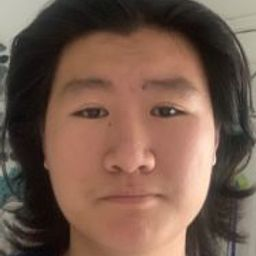🤔 Find out what you don't know with free Quizzes 🤔Start Quiz Now!# A wire of density $\delta(x, y, z)=15 \sqrt{y+2}$ lies along the curve $\mathbf{r}(t)=\left(t^{2}-1\right) \mathbf{j}+$ $2 t \mathbf{k},-1 \leq t \leq 1 .$ Find its center of mass. Then sketch the curve and center of mass together.

Integrals

Vectors

Vector Functions

### Discussion

You must be signed in to discuss.
##### Top Calculus 3 Educators##### Catherine R.

Missouri State University##### Kristen K.

University of Michigan - Ann Arbor

Lectures

Join Bootcamp

### Video TranscriptUniversity of California, Berkeley

#### Topics

Integrals

Vectors

Vector Functions

##### Top Calculus 3 Educators##### Catherine R.

Missouri State University##### Kristen K.

University of Michigan - Ann Arbor

Lectures

Join Bootcamp